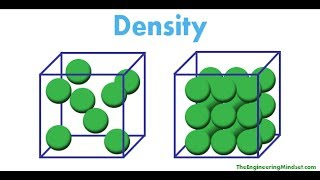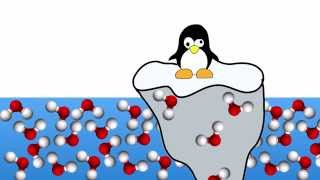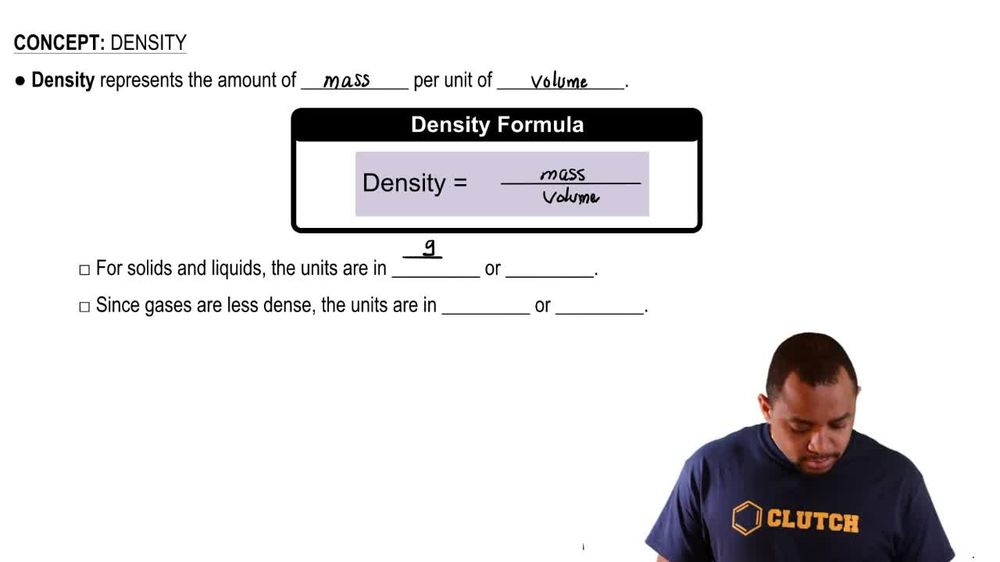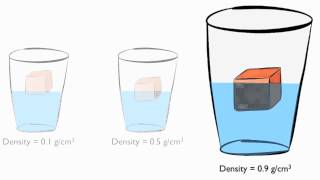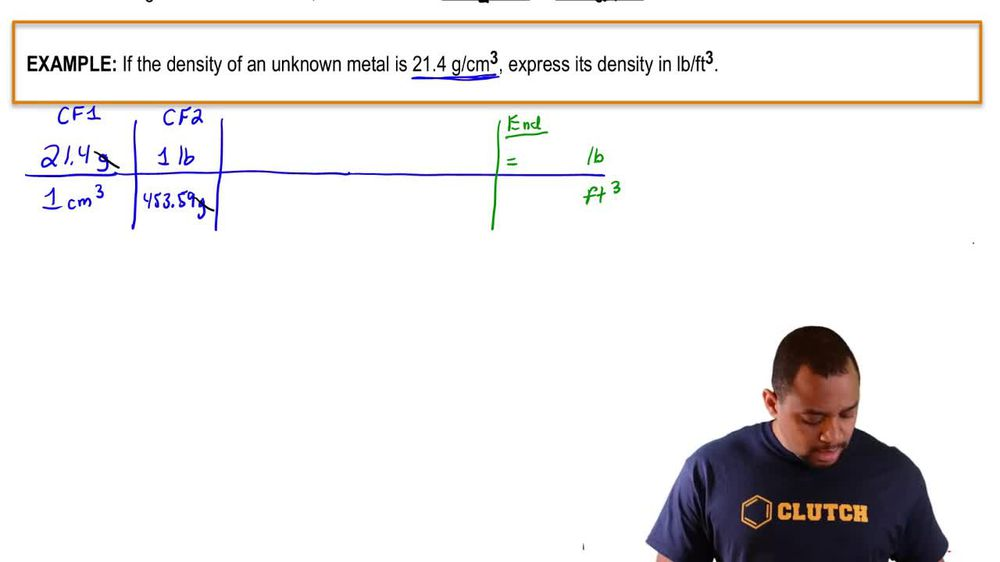Start typing, then use the up and down arrows to select an option from the list.
1. 1. Intro to General Chemistry2. Density
Problem

# When an irregularly shaped chunk of silicon weighing 8.763 g was placed in a graduated cylinder containing 25.00 mL of water, the water level in the cylinder rose to 28.76 mL. What is the density of silicon in g/cm3?

Relevant Solution1m
Play a video:
Hey everyone. So here we're told an empty 200 ml graduated cylinder weighs 243 g. It was filled with an unknown liquid up to 160 ml mark And now weighs 289 g. Now we need to calculate the density of the unknown liquid in terms of grams per mil leader. Alright, so When it's empty it weighs 243 g. When I pour the unknown liquid, it becomes to 89 g. So what we're gonna do here is we're going to subtract these two numbers from each other. So 29 g -243 g. That gives me 46 g as the mass of the liquid. Okay, now remember density is equal to mass over volume. So we have the 46 g so far. Now that we've found the mask, what do we do to figure out the volume? So look back on the question, where can we find the volume? Yeah. So if we look here, it says that we pour to the 160 ml mark. So that means we poured in 160 mls of our unknown liquid. That's my volume. So when I plugged that in, I'm gonna get .2 875 g per milliliter which rounds approximately here, a little bit off. But here the closest answer would be a option. A So option A would be our final answer